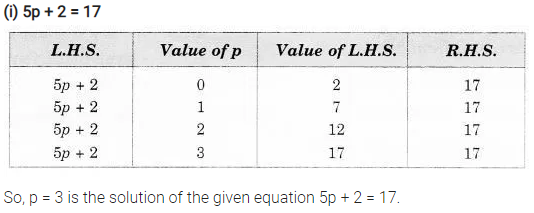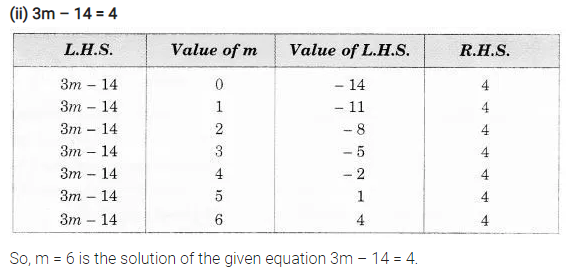# Solve the following equations by trial and error method. 5p + 2 = 17

Solve the following equations by trial and error method.

1. 5p + 2 = 17
2. 3m – 14 = 4.